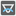## Question related to missionThe Angles of a Triangle

I would like to give some feedback about ...

From: http://www.checkio.org/mission/triangle-angles/solve/

HTTP\_USER\_AGENT:

Mozilla/5.0 (Windows NT 6.1; WOW64) AppleWebKit/537.36 (KHTML, like Gecko) Chrome/36.0.1985.125 Safari/537.36

My Code:

def checkio(a, b, c):
import math
#finding the first angle using the law of cosines.
#if an error is thrown it is because the side lengths given do not form a triangle
try:
angleC = math.acos(((a ** 2) + (b ** 2) - (c ** 2))/(2 * a * b))
except:
angleA, angleB, angleC = 0, 0, 0
if angleC != 0: #we only need to solve for the other angles if angleC was found
#now that we have one angle, we can use the law of sines to find the second angle
angleB = math.asin((math.sin(angleC) * b) / c)

#converting the first two angles to degrees since the math module uses radians
angleC = round(math.degrees(angleC))
angleB = round(math.degrees(angleB))

#now that we have 2 angles, we can easily find the third since all three angles will add up to 180 degrees
angleA = 180 - (angleC + angleB)

#now we just return the angles
return [angleA, angleB, angleC]

#These "asserts" using only for self-checking and not necessary for auto-testing
if __name__ == '__main__':
assert checkio(4, 4, 4) == [60, 60, 60], "All sides are equal"
assert checkio(3, 4, 5) == [37, 53, 90], "Egyptian triangle"
assert checkio(2, 2, 5) == [0, 0, 0], "It's can not be a triangle"

My code fails for checkio(5, 4, 3), which I am told should be [37, 53, 90]. The only problem is the biggest angle should be opposite the longest side, correct? Plus this is the exact same answer given in the asserts for checkio(3, 4, 5) above. Am I missing something?7